Refer to our Texas Go Math Grade 7 Answer Key Pdf to score good marks in the exams. Test yourself by practicing the problems from Texas Go Math Grade 7 Module 1 Quiz Answer Key.

1.1 Rational Numbers and Decimals

Write each mixed number as a decimal.

Question 1.
4$$\frac{1}{5}$$ __________
First. write $$\frac{1}{5}$$ as a decimal.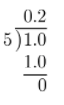Then, add 4 to the result.
4 + 0.2 = 4.2

Question 2.
12$$\frac{14}{15}$$ _________
First. write $$\frac{14}{15}$$ as a decimal.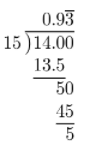Then, add 12 to the result.
12 + $$0.9 \overline{3}$$ = $$12.9 \overline{3}$$

Question 3.
5$$\frac{5}{32}$$ ___________
First. write $$\frac{5}{32}$$ as a decimal.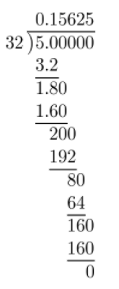Then, add 5 to the result.
5 + 0.15625 = 5.15625

1.2 Relationships Between Sets of Numbers

Question 4.
Are all whole numbers rational numbers? Explain.
True Every whole number is included in the set of integers, and every integer is incLuded in the set of rational numbers.
Whole numbers are a subset of the set of rational numbers

Find each sum.

Question 5.
4.5 + 7.1 = _____________
11.6

Question 6.
5$$\frac{1}{6}$$ + (-3$$\frac{5}{6}$$) = ____________
Write mixed fractions as proper fractions.1.4 Subtracting Rational Numbers

Find each difference.

Question 7.
–$$\frac{1}{8}$$ – (6$$\frac{7}{8}$$) = _____________
= -6$$\frac{8}{8}$$
= -7

Question 8.
14.2 – (-4.9) = ___________
= 14.2 + 4.9
= 19.1

1.5 Multiplying Rational Numbers

Multiply.

Question 9.
-4($$\frac{7}{10}$$) = _______________Question 10.
-3.2(-5.6)(4) = ___________
The result will be positive, because the expression has an even number of negative signs.
= 3.2(5.6)(4)
= 17.92(4)
= 71.68

1.6 Dividing Rational Numbers

Find each quotient.

Question 11
–$$\frac{19}{2}$$ ÷ $$\frac{38}{7}$$ = _______________
= -8 ÷ $$\frac{38}{7}$$
Write using multiplication:
= -8 × $$\frac{7}{38}$$
= – $$\frac{28}{19}$$ (= -1$$\frac{9}{28}$$)

Question 12.
$$\frac{-32.01}{-3.3}$$ = ___________
The quotient will be positive because the signs are the same.
Write decimal numbers as fractions:
$$\frac{\frac{3201}{100}}{\frac{33}{10}}$$
Write the complex fraction as division:
$$\frac{3201}{100}$$ ÷ $$\frac{33}{10}$$
Write using multiplication:
$$\frac{3201}{100}$$ $$\frac{10}{33}$$ = $$\frac{97}{10}$$
= 9.7

Essential Question

Question 13.
How can you use rational numbers to represent real-world problems?
We can use rational numbers to represent real-world problems by expressing some kind of ratio.
E g
Martha planted 30 flowers, 24 of them did not wither. Her success rate was $$\frac{24}{31}$$.

Texas Go Math Grade 7 Module 1 Mixed Review Texas Test Prep Answer Key

Selected Response

Question 1.
What is -7$$\frac{5}{12}$$ written as a decimal?
A. -7.25
B. -7.333.
C. -7.41666…
D. -7.512
C. -7.41666…

First, write $$\frac{5}{12}$$ as a decimal.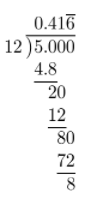Then, add 7 to the result.
7 + $$0.41 \overline{6}$$ = $$7.41 \overline{6}$$
Now, since the starting number was negative, this one has to be negative too.
-7$$\frac{5}{12}$$ = –$$7.41 \overline{6}$$

Question 2.
Which set or sets does the number -9$$\frac{1}{2}$$ belong to?
A. Integers only
B. Rational numbers only
C. Integers and rational numbers only.
D. Whole numbers, integers, and rational numbers
B. Rational numbers only

-9$$\frac{1}{2}$$ is a mixed fraction. Thus, it is onLy a rational number.

Question 3.
Renee ate $$\frac{1}{4}$$ of a pizza, and Sumi ate of the same pizza. How much of the pizza did they eat in all?
A. $$\frac{1}{7}$$ of the pizza
B. $$\frac{2}{7}$$ of the pizza
C. $$\frac{5}{12}$$ of the pizza
D. $$\frac{7}{12}$$ of the pizza
D. $$\frac{7}{12}$$ of the pizza

We have to add how much Renee ate, and how much Sumi ate.
$$\frac{1}{4}$$ + $$\frac{1}{3}$$ = $$\frac{3+4}{12}$$
= $$\frac{7}{12}$$
Renee and Sumi ate $$\frac{7}{12}$$ of the pizza

Question 4.
Kareem had $25 in his bank account on Monday. The table shows his account activity for the next four days. What was the balance in Kareem’s account on Friday?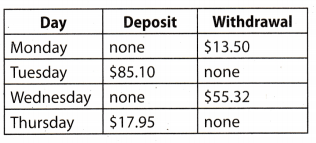A.$59.23
B. -$9.23 C.$9.23
D. -$59.23 Answer: A.$59.23

Use positive numbers to represent deposit and negative numbers to represent withdrawal. Then, add it up to account’s balance before any deposits or withdrawals, 25.
25 + (-13.50) + 85.10 + (-55.32) + 17.95 = 25 + 85.10 + 17.95 – 13.50 – 55.32
= – 110.10 + 17.95 – 13.50 – 55.32
= 128.05 – 13.50 – 55.32
= 114.55 – 55.32
= 59.2:3
The balance in Kareem’s account. on Friday was $59.23. Question 5. A used boat is on sale for$2,400. Austin makes an offer equal to of this price. How much does Austin offer for the boat?
A. $3,600 B.$1,600
C. $1,800 D.$800
C. $1,800 Start by multiplying 2400 and $$\frac{2}{3}$$. 2400 × $$\frac{2}{3}$$ = 1600 Austin offers$1600.

Question 6.
Working together, 9 friends pick 23 bags of apples at an orchard. They divide the bags of apples equally between them. How many bags does each friend get?
A. 32$$\frac{2}{5}$$ bags
B. 14$$\frac{2}{5}$$ bags
C. 2$$\frac{3}{5}$$ bags
D. 2$$\frac{5}{9}$$ bags
C. 2$$\frac{3}{5}$$ bags

Start with dividing 23$$\frac{2}{5}$$ by 9:
23$$\frac{2}{5}$$ ÷ 9
Write mixed fraction as proper fraction:
$$\frac{117}{5}$$ ÷ 9
Write using multiplication:
$$\frac{117}{5}$$ × $$\frac{1}{9}$$ = $$\frac{13}{5}$$
= 2$$\frac{3}{5}$$
Each friend gets 2$$\frac{3}{5}$$ bags

Question 7.
The Flathead Rail Tunnel in Montana is about 7$$\frac{3}{4}$$ miles long. A train travels at a speed of $$\frac{3}{4}$$ mile per minute. How long will it take the train to go through the tunnel?
A. $$\frac{7}{16}$$ minute
B. 5$$\frac{3}{16}$$ minutes
C. 8$$\frac{1}{3}$$ minutes
D. 10$$\frac{1}{3}$$ minutes
D. 10$$\frac{1}{3}$$ minutes

Start with diving by 7$$\frac{3}{4}$$ by $$\frac{3}{4}$$.
Write mixed fraction as proper fraction:
$$\frac{31}{4}$$ ÷ $$\frac{3}{4}$$
Write using multiplication:
$$\frac{31}{4}$$ × $$\frac{4}{3}$$ = $$\frac{31}{3}$$
= 10$$\frac{1}{3}$$
The train will 10$$\frac{1}{3}$$ minutes to pass the tunnel.

Gridded Response

Question 8.
What is the value of (-2.75 )(-1 .16)?